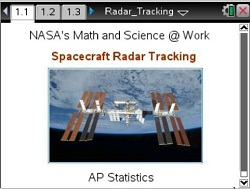# Activities

••• ##### Subject Area

• Math: Statistics: Data Analysis
• Math: Statistics: Probability and Random Variables

• ##### Author9-12

• ##### Device
•TI-Nspire™ CX/CX II
•TI-Nspire™ CX CAS/CX II CAS
• ##### Software

TI-Nspire™
TI-Nspire™ CAS

3.2

• ##### Report an Issue#### Activity Overview

In this lesson, students will calculate and interpret the meaning of the correlation coefficient and coefficient determination.

#### Objectives

In this lesson, students will:

• calculate and interpret the meaning of the correlation coefficient, r, and the coefficient of determination, r2.
• write a regression equation, and interpret the meaning of the slope and y-intercept in context to the problem.
• make predictions based on a least squares regression model
• plot residuals, and interpret their graphical form.

#### Vocabulary

• correlation
• coefficient
• regression equation